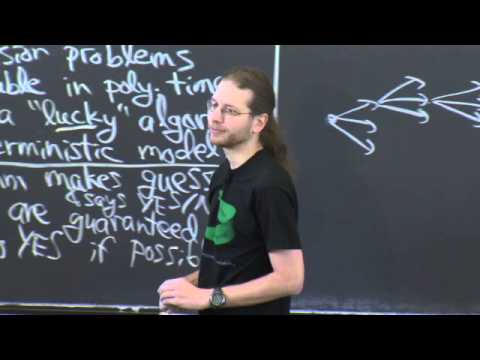# MIT OpenCourseWare► Play all

# MIT 6.006 Introduction to Algorithms, Fall 2011

This course provides an introduction to mathematical modeling of computational problems. It covers the common algorithms, algorithmic paradigms, and data structures used to solve these problems. The course emphasizes the relationship between algorithms and programming, and introduces basic performance measures and analysis techniques for these problems.
This course provides an introduction to mathematical modeling of computational problems. It covers the common algorithms, algorithmic paradigms, and data structures used to solve these problems. The course emphasizes the relationship between algor...